## Su Doku

Su Doku is usually played on a 9 by 9 board, divided into 3 by 3 cells. You can generalize this into playing on an M×M square board broken into non-overlapping rectangular cells, each containing M squares (an obvious question is to how define a puzzle where M is a prime number). The solution of the puzzle is to place M symbols on the board such that each row, column or cell contains each symbol exactly once, without moving the initial clues. Puzzles with M<9 are called Sub Dokus, those for M>9 are called Super Dokus.

Technorati tag:

Related topic: Kakuro

## Shi Doku counting

 Count of Total ED* Solutions 288 2 Puzzles 13579680 4710 Irreducibles 85632 36 6-clue 1536 1 5-clue 58368 22 4-clue 25728 13
*: Essentially different — Two things are essentialy different under symmetries if they are not transformed into each other by symmetry.

Solutions are completed grids

Puzzles are proper puzzles, ie, those with an unique solution

Irreducibles are proper puzzles in which removal of any clue makes the puzzle improper

# Shi Doku

## History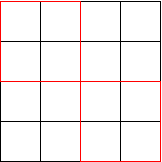The name Shi Doku comes from Japanese and means "four singles". Shi Doku was invented on Wednesday by someone who wanted a puzzle which could be solved while waiting for the lights to turn green. The puzzle is set on a four by four board divided into non-overlapping two by two cells. The object is to place the symbols "B" "O" "R" "E" on the board so that each row, column or cell contains each symbol exactly once. The puzzle starts with a set of symbols (called "clues") already placed on the board, which may not be moved.

Those who call this game Shin Doku are making an obscure joke in Japanese. Others have been known to carp that a boundary is a less strenuous version of "four singles".

## Some counting

### How many different Shi Doku solutions are possible?There are $4!=24$ possible arrangements of symbols in the upper left cell. For each of these the following analysis holds. So perform the analysis for the configuration shown in the figure. The total number of solutions is the result of the subsequent counting multiplied by 4!.Next we turn to the upper right cell. The upper row of this must contain the elements of the lower left row of the starting cell, that is {"R", "E"}, in either order. Similarly, the lower row must contain the elements of the upper row of the starting cell in either order. This gives 4 possible arrangements of the upper right cell. One such arrangement is shown on the right.

A similar argument can be framed for the lower left cell in terms of columns of the starting cell. This gives 4 possible arrangements of this cell for each arrangement of the starting cell and the upper right cell. The bottom right cell must now be completely determined.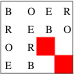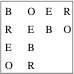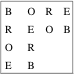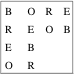These grids cannot be completed. The red squares on the first grid are positions where no symbol is allowed. The other three are easy to work out.

So for each arrangement of the top left cell, we seem to have 16 arrangements of the remainder. But that's not quite correct. Enumerating all possibilities shows that the 4 possibilities shown above are not allowed. This leaves 12 arrangements of all cells given an arrangement of the top left cell.

Thus the total number of Shi Doku solutions is $4!.12=288$. (An alternative proof is given in the documentation on the sequence A107739.) If you think this number is not mind bogglingly large, then just look at it in binary: it is 100100000. In comparison, the number of Su Doku solutions (in appropriately chosen base) is 10, as is the number of Super Doku boards.

The really interesting thing about these puzzles is not that the number is so large, but that it is actually very small. If one plays Shi Doku with the "Rule of the Jungle", ie, anything goes, then there are $416=4294967296$ states. This is the number of ways of placing any one of 4 symbols in 16 squares. The rules of the game select about one in 108. In Su Doku the Rule of the Jungle allows $981$ solutions, of which less than one in 1077 remain alive when the laws come marching in. The counting of Su Doku solutions (due to Felgenhauer and Jarvis, and, independently, Russell) therefore starts off being very similar to the counting above, but has to use many more tricks and intermediate theorems to complete.

### What is the maximum number of clues?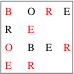We will count a valid puzzle as one in which the clues lead to an unique answer. Then the maximum number of clues is $16-3=13$. The picture on the right shows a situation in which 12 clues do not lead to a solution and a 13th is necessary. The analogous result is well known in Su Doku and is also true for all Super Dokus. Here is the result:
In a M×M Super Doku, the maximum number of clues is $M2-3$.

#### Independent clues

A set of clues is called independent if none of them can be derived from the other. (When this set is as large as it can get, then the remainder should be called derivable clues). The counting above does not contain independent clues. As you can see from the figure above, the clues in red make up one set of independent clues from which all the remainder can be derived. Thus, the example above needs 5 independent clues.

Challenge 1: What is the maximum number of independent clues possible? For Shi Doku the example above is maximal; the maximum number of independent clues possible is $5+1=6$. What is it for a general Super Doku?

In the example above, a different set of independent clues would be to take the clues as given in the left two columns, but in the right two columns use the two "E"s instead of the two "R"s. This tells us that two different sets of independent clues can lead to the same solution. Therefore, the counting of the previous section gave the number of solutions, not the number of possible puzzles.

Challenge 2: Count the number of different puzzles for Shi Doku. Two puzzles should be counted as different if the clues are different, even if they lead to the same solution. (The solution of this problem is solved given in the section the complete puzzle forest.)

### A different way to count

The previous way of counting deliberately followed closely the scheme developed by Felgenhauer and Jarvis to count the number of solutions in 9×9 Su Doku. Now we show an alternate way to accomplish the same counting which has some advantages. We name this method after a story by Jorge Luis Borges.

#### Garden of forking paths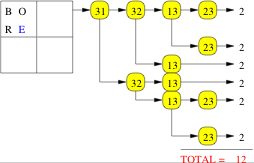First take one of the $4!$ ways of filling in the top left cell. A canonical choice is shown in the figure. (Note that only three of the four letters are independent clues, since the fourth, coloured blue in the figure, can be derived from the rest.) Next treat the remainder of the counting problem through a depth first enumeration. This works by trying to solve the Shi Doku completely, and whenever there is a choice, taking all the possible choices in turn. Applying this procedure recursively generates a branching tree of possibilities. We count the number of times a completed grid is reached by the tree.

Since the enumeration is exhaustive, there are no "missing" configurations. But how do we know that all the completed grids are different from each other, and that they should all be counted? First of all, at each step we make sure that we have "irreducible" choices, by eliminating all possibilities which can be eliminated at that point. This is built into the enumeration, and makes sure that there are no erroneous branches taken which are really the same. Then two configurations can only be the same if they can be taken into each other by symmetry operations. We guard against this by choosing a starting point which allows no symmetry operations. Thus the counting is both unique and exhaustive.

The result is illustrated above. Each oval indicates the position at which a choice is available: the symbol (ij) denotes that the choice is to be made in the square on the i-th column and j-th row. A completed solution is reached 12 times, giving 288 solutions as before.

#### Why this way?

Depth first enumeration has better run time in general than elimination of the kind used in 9×9 Su Doku, because non-productive branches are either eliminated as early as possible, or are never seen as an alternative. This happens in the two branches in the tree above where one goes directly from the choice in the square 13 to full solutions without going through the spurious choice in the square 23. An useful by-product of the depth-frist search is an automatic generation of maximum Su Dokus, as we argue below.

#### Maximum Shi Doku

The depth first enumeration gives the maximum number of clues for the following reason. The first three clues are clearly independent. The remaining clues are placed each time the search tree branches: each such clue is independent by definition. The maximum number of clues is obtained in the branch(es) of maximum depth— 7 for Shi Doku. This is almost a proof. The one step missing is to prove that another construction of the tree would not generate a different maximum: for example, if we took as the starting configuration BORE written across the first row, would we get the same result?

### What is the minimum number of clues?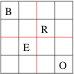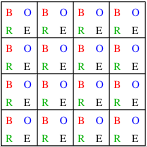A search gives the answer 4. An example is shown on the left. We now set out a counting procedure which seems to lead to the conclusion that the number cannot be further reduced. To set out the logic, we use the work sheet shown on the right. Every square has all possibilities penciled in; possibilities which are ruled out by a clue are removed as we proceed.1 It doesn't really matter where we place the first clue, and what it is. So we might as well place a "B" in the upper left corner. See how this rules out "B" from the shaded region: three squares across, three down, and the diagonal bulge. If the position of the clue were to be changed, then the shape of this region would change, but not the number of squares in the region. We will check how such regions intersect each other.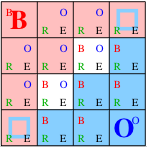2 For maximum mileage from the second clue it should be placed in a different row, column and cell from the first. Then the diagonal bulges of the two cannot overlap, and the only overlap is due to the intersection of the rows and columns. In this example, these intersections are at the two remaining corners of the board. For the maximum gain in these corners, the symbol in the second clue has to be different from the first; here we write in an "O". The number of white squares now reduces to two. The total number of symbols left in the shaded squares is 30. Since moving the second clue so that it overlaps with the first either in row, column or cell increases the number of white squares without reducing the number of symbols in the shaded squares, we know that would not be an optimal second move.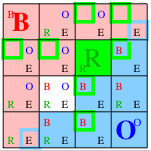3 If we place the third clue in one of the white squares then the number of white squares remaining is one. The number of white squares can be reduced if this clue is placed in one of the diagonal bulges; but this would mean that the diagonal bulge of the new clue is eaten up by one of the old clues. In the configuration shown, the diagonal bulge is crucial, since it determines one corner: the upper right in the example shown here. Of course, the third clue has to be different symbol from the first two for this to happen: here we choose to make it a "R".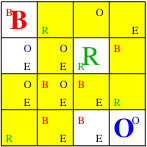4 This corner's effects ripple back, as shown by the yellow squares in the picture on the right. Among the effects are a complete solution of the first row and the last column. Once these groups are determined every square has been modified. Next there is a wide choice of a fourth clue which completes the construction of the puzzle. In the example given at the top of this section we have chosen to place this last clue in such a way as to make the puzzle look symmetric.

The main idea in this construction is to place the four clues in independent rows, columns and cells. A fortunate circumstance that helped us was that the number of symbols (4) was one more than the number of groups which had to have each number once (ie, rows, columns and cells). As a result, after placing 3 clues the ripple back effects of step 4 started. For any other Super Doku this will happen later. The best statement that we can make is the following:
In a M×M Super Doku the minimum number of clues has to be at least M-1.

Here is the earliest reference to 4 clue Shi Doku. Here is the proof that 3 clues do not suffice.

Challenge 3: Prove an upper bound on the minimum number of clues for Su Doku or any Super Doku. For that matter, prove the lower bound in the statement above. (For 6×6 grids, 9 clues seems to suffice. For 9×9, standard sudoku, it is claimed that 17 clues are enough.)

Challenge 4: Improve the both the bounds for any Super Doku. The construction in Shi Doku given above is not a proof. It could help in the general solution if a proof could be produced.

### The complete puzzle forest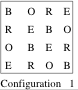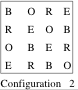Essentially different solutions are those which cannot be transformed into each other by some symmetry of permutations of the letters, and some transformation of the empty grid. There are two essentially different solutions for Shi Doku, as shown above. All questions about puzzles can be answered by examining all the puzzles which give rise to each of these solutions. For reasons explained elsewhere this construction is called the puzzle forest. The puzzle forest for Shi Doku specified below was obtained by Ed Russell and me.

 Clues Puzzles (C1) Puzzles (C2) Irreducible (C1) Irreducible (C2) 16 1 1 15 16 16 14 120 120 13 560 560 12 1812 1816 11 4272 4320 10 7480 7744 9 9696 10560 8 9064 10890 7 5760 8272 6 2209 4320 16 5 416 1280 256 176 4 12 128 12 128 Total 41401 50027 284 304

This enumeration shows that the number of Shi Doku puzzles is immensely larger than the number of solutions. This is true even if we count only the irreducible puzzles, which are those that no longer have an unique solution is any one clue is removed. Second, it shows that the minimum number of clues is 4. Finally it shows that the maximum number of clues in an irreducible puzzle is 6, and such puzzled only have as solution the configuration 1. Every 6 clue puzzle which has configuration 2 as the solution is reducible.

### How many satisfactory puzzles?

In the literature on Su Doku one finds references to bifurcation; also called Ariadne's thread, but more commonly known as trial and error. A solution by this means leaves me feeling a little unsatisfied: I am carrying out a set of instructions that any computer can be programmed to execute (the algorithm is known in computer science as depth-first search). So where is the fun which I expected out of solving a puzzle?

Challenge 5: Define "satisfactory" precisely in a sense that a large fraction of Su Doku addicts would agree to. Count the number of satisfactory puzzles. The London Times puzzle claims not to need trial and error: if so the definition of "satisfactory" should encompass all possible puzzles that could be published in this newspaper; ie, the program that generates these puzzles should be provably in the class that you define.

While doing the enumeration of Shi Doku puzzles I found that depth-first search is unnecessary for these puzzles. It is enough to use "pencil marks" and go over the grid repeatedly, eliminating numbers which already appear in the same row, column or block as the cell in question. Thus, all Shi Doku puzzles are satisfactory. This is an exhaustive enumeration, and hence not an elegant proof. (My definition of an elegant proof is that it should throw light on at least one thing other than the theorem being proven.)

© Sourendu Gupta. Mail me if you want to reproduce any part of this page. My mailing address is a simple (satisfactory) puzzle for you to solve. Created on 27 August, 2005. Last updated on 04 March, 2006.